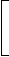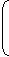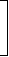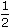# Aptitude - True Discount - Discussion

### Discussion :: True Discount - General Questions (Q.No.14)

14.

The present worth of Rs. 1404 due in two equal half-yearly installments at 8% per annum simple interest is:

 [A]. Rs. 1325 [B]. Rs. 1300 [C]. Rs. 1350 [D]. Rs. 1500

Explanation:

Required sum = P.W. of Rs. 702 due 6 months + P.W. of Rs. 702 due 1 year hence
= Rs.100 x 702+100 x 702100 + (8 x 1)100 + 8 x= Rs. (675 + 650)
= Rs. 1325.

 Sara said: (May 17, 2011) Can you please tell in the second bracket why did you multiply 8x1 ?

 Harshitha said: (Jun 10, 2011) Formula for sum=P*100/(100+(T*R) This is nothing but derivation from simple interest = (P*T*R)/100 .

 Adi said: (Aug 13, 2011) Can you please explain me the solution of above question ?

 Dileep said: (Feb 1, 2012) Since 8*(12/12)=8*1 8*(6/12)=8*(1/2)

 K N Chethan said: (Sep 6, 2012) What is 100*702/(100+8*1/2)?

 Rohit said: (May 19, 2013) Two equal half yearly installments?

 Rahul said: (Sep 29, 2013) Shouldn't it be 1/2 both the times?

 Kiranmayi Paila said: (Jan 4, 2015) First half year includes months i.e. 1/2 but second half early = 6+previous 6 months i.e. 1/2+1/2 = 1.

 Pavithra said: (Jan 29, 2015) Can you please tell the calculation of that?

 Girish said: (Aug 2, 2015) Why do we use 8 over there?

 Tejaswini said: (Aug 21, 2015) Though simple interest formula is p*t*r/100 why there is complexity in calculation can you please explain it in detail?

 Niharika said: (Jun 1, 2016) @Tejaswini. S.I = p * t * r/100. A = P + S.I. = P + P * T * R/100. = P(1 + T * R/100). = P(100 + T*R/100). So, P = (100 * A/100 + T * R).

 Ramesh said: (Jul 19, 2016) Why the interest can't be 4% each time?

 Bhavani said: (Jan 23, 2017) May, I know how 675 came?

 Bhavani said: (Jan 23, 2017) Can anyone explain how the calculation is done?

 Srishti said: (Jan 9, 2018) What is the formula used here? Please tell me.

 Srishti said: (Jan 9, 2018) Why 1yr is used in second bracket?

 Vikas said: (Jan 12, 2018) Yeah, it should be 1/2 even in the second bracket I guess because it is the equal half yearly basis.

 Sravani said: (Mar 12, 2018) hi, In the above problem first half-yearly 702 and second half yearly 702+first half yearly i.e., 1year so in the second bracket, it is taken as one year. A=p+S.I. A=P+(PTR/100), A=P(1+TR/100), SO Coming To The Problem, for first half-yearly, (100*702/100+8*1/2), For second Half Yearly, (100*702/100+8*1).

 Rajesh said: (May 16, 2018) Since it is in SI, only that interest part will be added in each 6 months. So, what is the need for 1year in the second part?

 Anonymous said: (Jul 8, 2018) Why second time we take only 702 if we consider time as 1 year?

 Pavan G J said: (Aug 21, 2020) See, first 702rs pw is calculated for 6 months and second 702rs pw is calculated for 1 year because 6months + 6months = 1year i.e., we have to calculate the present worth in present the question is like divide the sum by 2 and calculate the pw of firtst half in 6 months and pw for 2nd half for 6 months. For 2nd half we should consider 1year because when you think wrt pw it becomes 1year. Hope all of you can get.